### Simple Exponential Smoothing

Suitable for forecasting data with no clear trend or seasonal pattern.

library(fpp2)
oildata <- window(oil, start=1996)
autoplot(oildata) +
ylab("Oil (millions of tonnes)") + xlab("Year")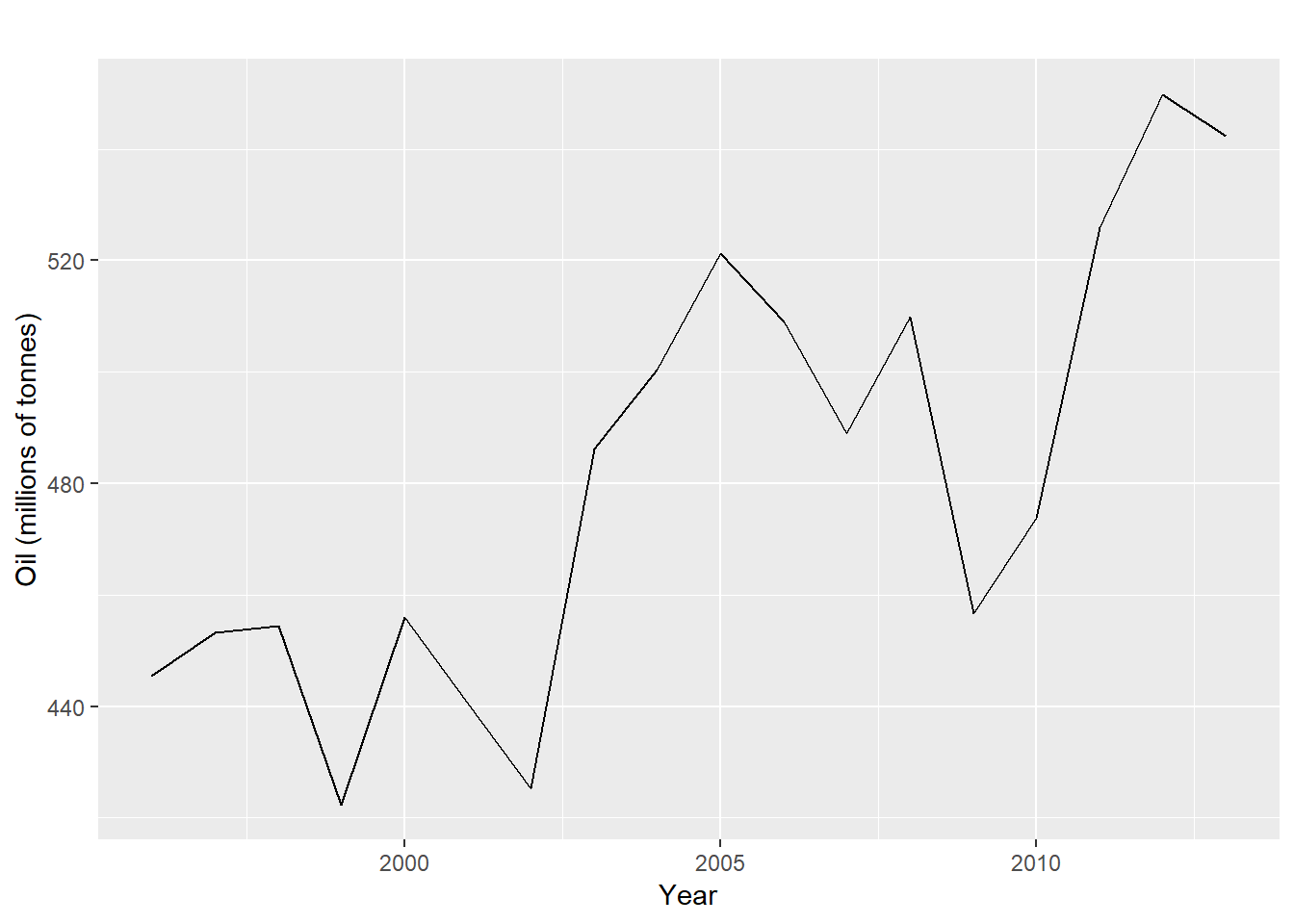SES is applied as

oildata <- window(oil, start=1996)
# Estimate parameters
fc <- ses(oildata, h=5)
# Accuracy of one-step-ahead training errors
round(accuracy(fc),2)
##               ME  RMSE   MAE MPE MAPE MASE  ACF1
## Training set 6.4 28.12 22.26 1.1 4.61 0.93 -0.03
fc
##      Point Forecast    Lo 80    Hi 80    Lo 95    Hi 95
## 2014       542.6806 504.4541 580.9070 484.2183 601.1429
## 2015       542.6806 492.9073 592.4539 466.5589 618.8023
## 2016       542.6806 483.5747 601.7864 452.2860 633.0752
## 2017       542.6806 475.5269 609.8343 439.9778 645.3834
## 2018       542.6806 468.3452 617.0159 428.9945 656.3667

Plotting it

autoplot(fc) +
autolayer(fitted(fc), series="Fitted") +
ylab("Oil (millions of tonnes)") + xlab("Year")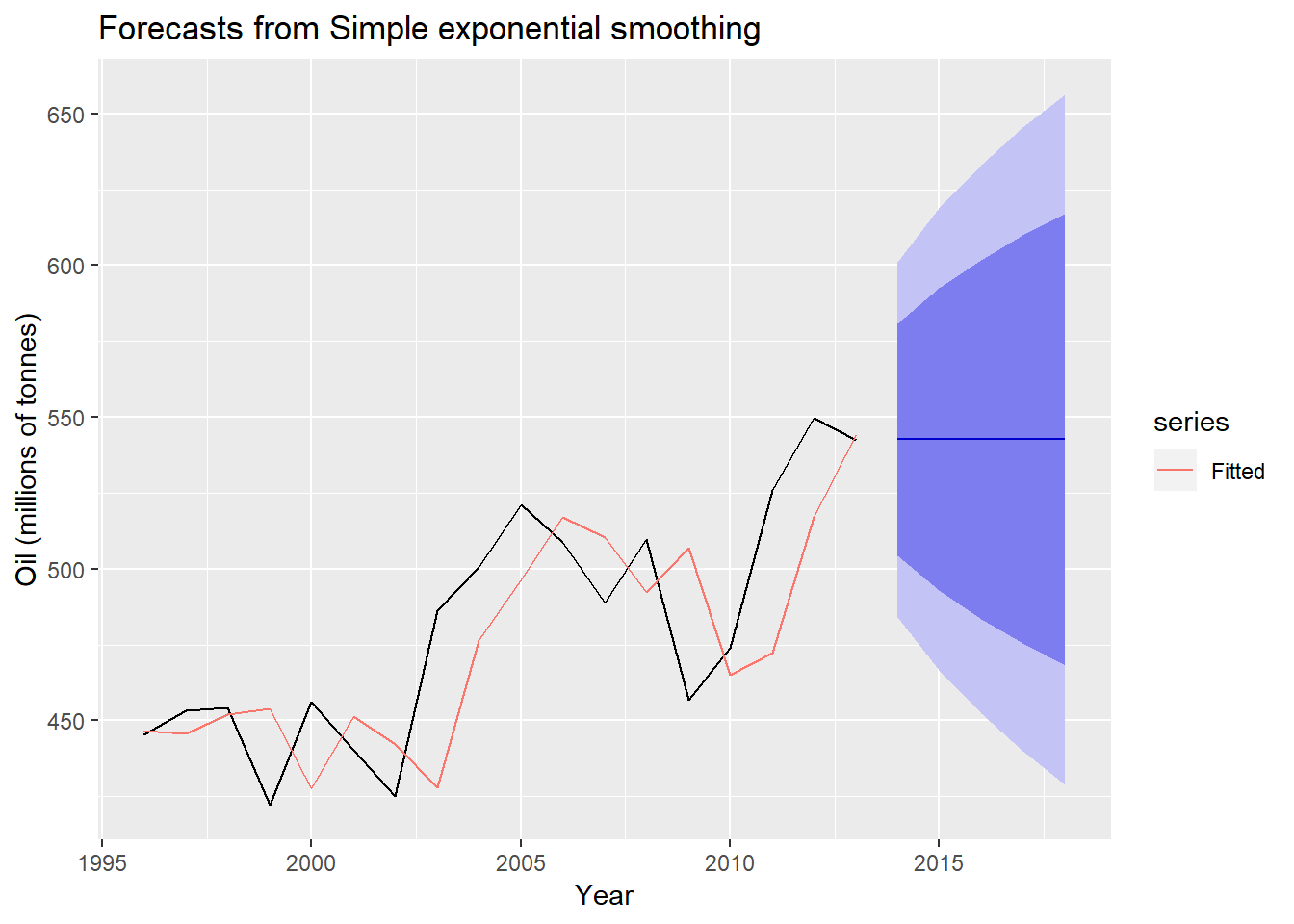The prediction intervals show that there is considerable uncertainty in the future values of oil production over the five-year forecast period.

## Holt’s Linear Trend Method

Holt (1957) extended simple exponential smoothing to allow the forecasting of data with a trend. This method involves a forecast equation and two smoothing equations (one for the level and one for the trend):

### Damped Trend Method

The forecasts generated by Holt’s linear method display a constant trend (increasing or decreasing) indefnitely into the future. Empirical evidence indicates that these methods tend to over-forecast, especially for longer forecast horizons. Motivated by this observation, Gardner & McKenzie (1985) introduced a parameter that “dampens” the trend to a flat line some time in the future.

eg. air passengers

air <- window(ausair, start=1990)
fc <- holt(air, h=15)
fc2 <- holt(air, damped=TRUE, phi = 0.9, h=15)
autoplot(air) +
autolayer(fc, series="Holt's method", PI=FALSE) +
autolayer(fc2, series="Damped Holt's method", PI=FALSE) +
ggtitle("Forecasts from Holt's method") + xlab("Year") +
ylab("Air passengers in Australia (millions)") +
guides(colour=guide_legend(title="Forecast"))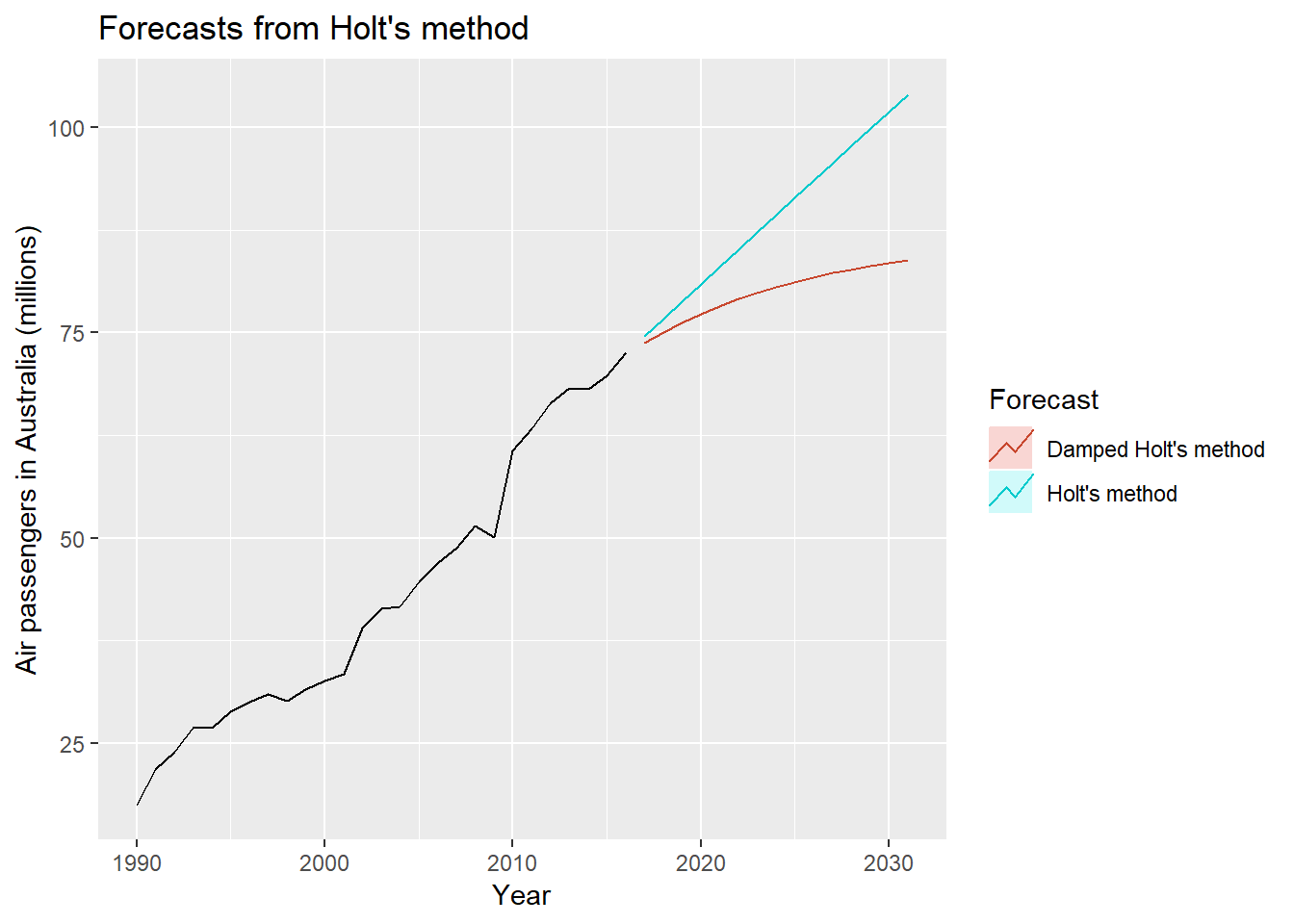Here phi is kept at 0.90. But the function calculates by itself.

eg.

fc <- holt(livestock,damped = TRUE)

Lets estimate the parameters

fc[["model"]]
## Damped Holt's method
##
## Call:
##  holt(y = livestock, damped = TRUE)
##
##   Smoothing parameters:
##     alpha = 0.9999
##     beta  = 3e-04
##     phi   = 0.9798
##
##   Initial states:
##     l = 223.35
##     b = 6.9046
##
##   sigma:  12.8435
##
##      AIC     AICc      BIC
## 427.6370 429.7370 438.7379

lets plot the forecast

autoplot(fc) +
xlab("Year") + ylab("Livestock, sheep in Asia (millions)")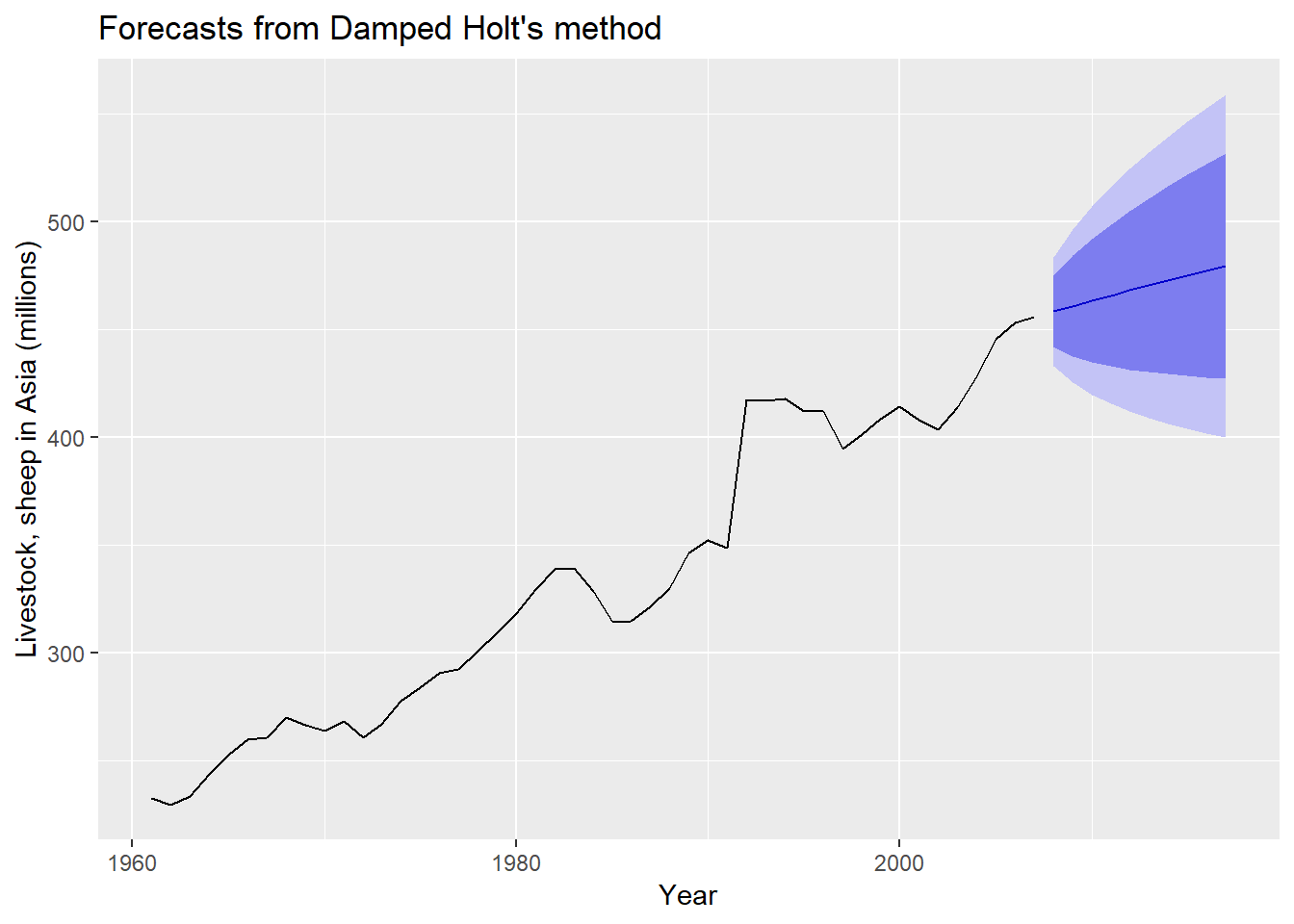## Holt-Winter’s seasonal Method

aust <- window(austourists,start=2005)
fit2 <- hw(aust,seasonal="multiplicative")
autoplot(aust) +
autolayer(fit1, series="HW additive forecasts", PI=FALSE) +
autolayer(fit2, series="HW multiplicative forecasts",
PI=FALSE) +
xlab("Year") +
ylab("Visitor nights (millions)") +
ggtitle("International visitors nights in Australia") +
guides(colour=guide_legend(title="Forecast"))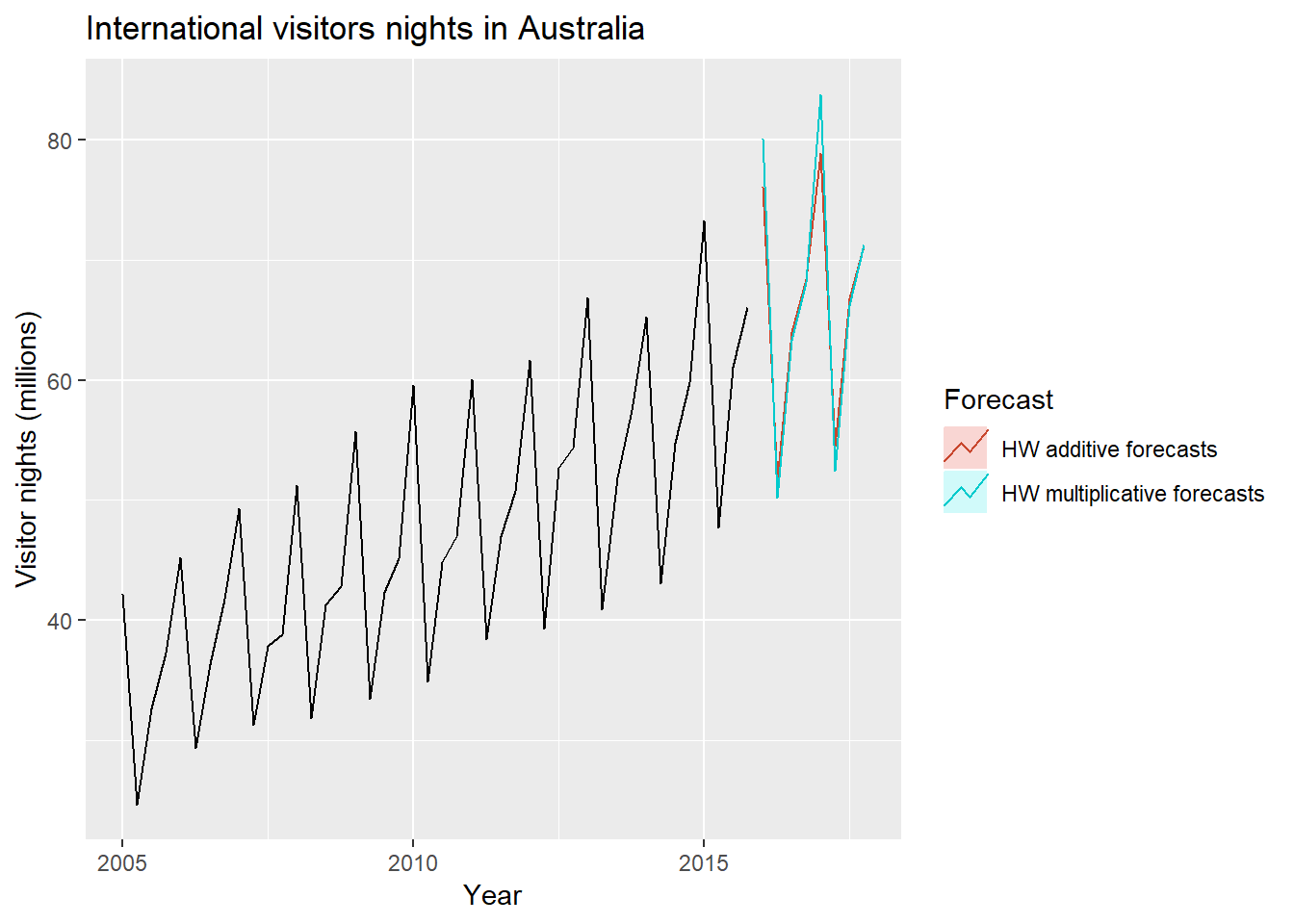We can also use hw damped method. It can be used for daily data.

fc <- hw(subset(hyndsight,end=length(hyndsight)-35),
damped = TRUE, seasonal="multiplicative", h=35)
autoplot(hyndsight) +
autolayer(fc, series="HW multi damped", PI=FALSE)+
guides(colour=guide_legend(title="Daily forecasts"))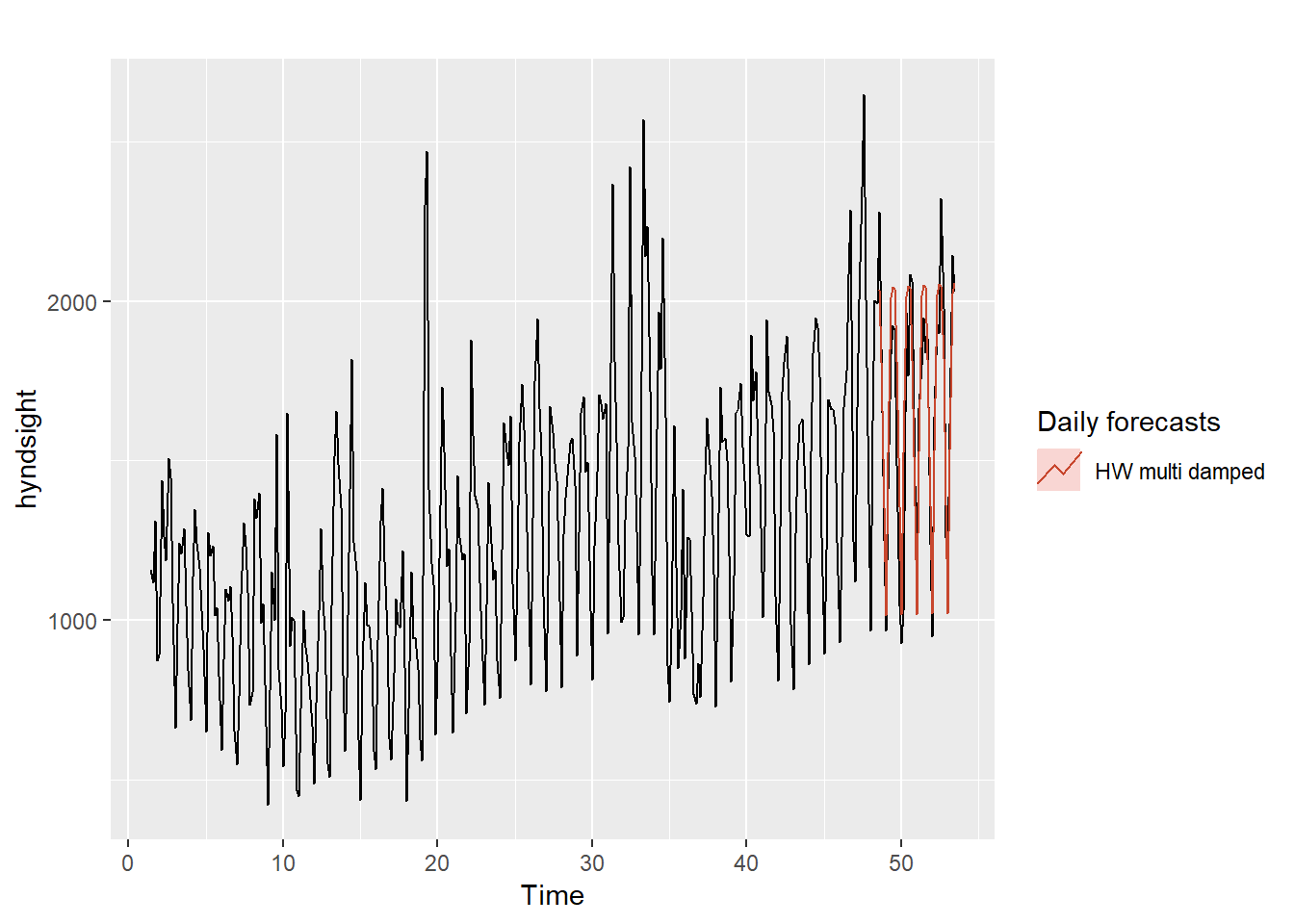save.image("workspace.Rdata")